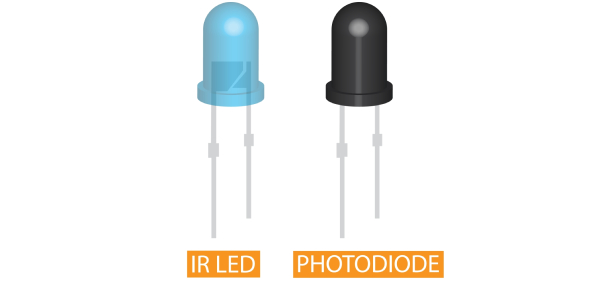# How Well Do You Know Photodiode Quiz

10 Questions | Attempts: 2544SettingsHow well do you know about photodiodes? Here, we have a quiz based on that. The aim of this photodiode quiz is to provide you with accurate knowledge of the concepts of special diodes. A photodiode is a light-sensitive semiconductor device that follows a P-N junction rule. It converts photons into electrical currents. What else do you know about it? Have you ever worked or used such diodes? Just take the quiz below and improve your understanding of photodiodes.

• 1.
When a photon of sufficient energy strikes the diode, it excites an electron, thereby creating a free electron. What is this phenomenon called?
• A.

Peltier Effect

• B.

Photoelectric Effect

• C.

Seebeck Effect

• D.

Dipole Effect

• 2.
By definition, N-type semiconductor has an excess of  _______ compared to the P-type region, which has an excess of ______ compared to the N-type region.
• A.

Electrons, holes

• B.

Holes, electrons

• C.

Holes, holes

• D.

Electrons, electrons

• 3.
When a diode is reverse biased, the width of the depletion layer __________, causing the capacitance to _________.
• A.

Increases, increase

• B.

Increases, decrease

• C.

Decreases, increase

• D.

Decreases, decrease

• 4.
Photodiodes operate by absorption of photons or charged particles and generate a flow of current in an external circuit, _______ to the incident power. The light is absorbed __________ with distance and is _________ to the absorption coefficient.
• A.

Proportional, exponentially, proportional

• B.

Proportional, logarithmically, inversely proportional

• C.

Inversely proportional, exponentially, unrelated

• D.

Inversely proportional, exponentially, proportional

• 5.
Dark current is
• A.

Produced by forward biasing the photodiode

• B.

Produced by the random generation of electrons and holes in the depletion region

• C.

Produced by incident photons generating electrons and holes in the depletion region

• D.

Produced by reversed biasing the photodiode

• 6.
The presence of dark current decreases the sensitivity of the photodiode to light.
• A.

True

• B.

False

• 7.
When the p–n junction is forward biased,
• A.

The electric charge remains the same as the junction barrier is not affected

• B.

The electric charge flow is restricted due to the increased junction barrier

• C.

The electric charge flows freely due to the decreased junction barrier

• D.

All of these statements are true.

• 8.
If a diode is reverse biased, the voltage at the anode is higher than at the cathode.
• A.

True

• B.

False

• 9.
Which can be used as a photo detector in Fiber Optic Communication?
• A.

PIN photodiodes

• B.

Tunnel diode

• C.

LED

• D.

LASER diode

• 10.
The light consists of small energy packets called _______ according to The Quantum Theory.
• A.

Neutron

• B.

Krypton

• C.

Photon

• D.

Proton

## Related TopicsBack to top
×

Wait!
Here's an interesting quiz for you.